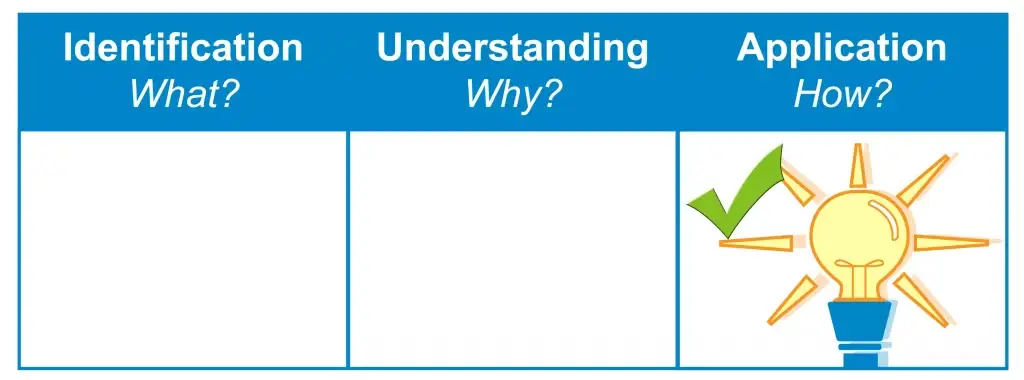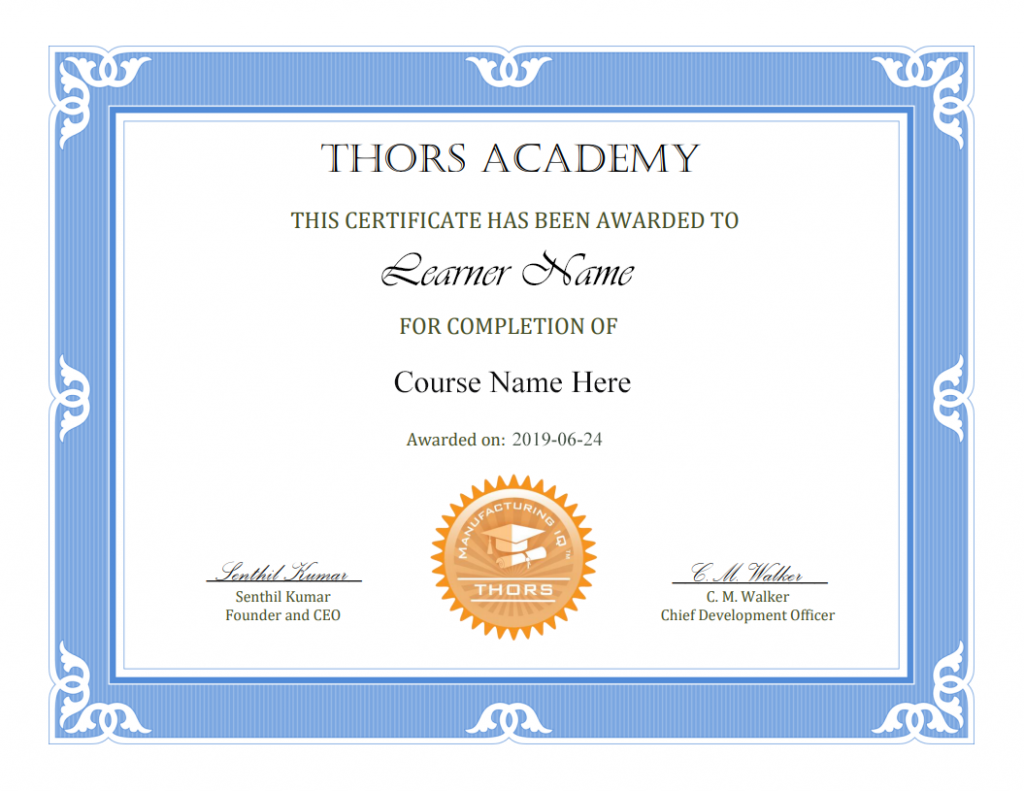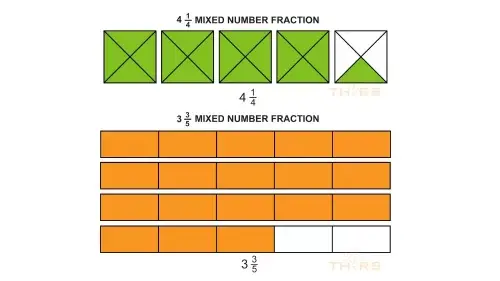THORS eLearning Solutions# Shop Math: Fractions

\$340.00

The Shop Math: Fractions course aims to provide learners with an introduction to the different types of fractions, how to reduce and convert fractions into a preferred form, and how to perform various operations using fractions.
Learning Hours: 4

## Course Description

The Shop Math: Fractions course aims to provide learners with an introduction to the different types of fractions, how to reduce and convert fractions into a preferred form, and how to perform various operations using fractions. Step-by-step examples, animations, and explanations are provided for each topic to further clarify how to solve simple and more complex problems. This course is valuable for both new learners and those returning to refresh their knowledge on fractions.

## Who will benefit from this Shop Math: Fractions course?

Personnel at an organization that are required to understand and use mathematical concepts to complete daily tasks.

Shop floor personnel.

## Course Classification*THORS uses the Bloom’s Taxonomy Methodology for our course development.

## Certificate Awarded for Shop Math: Fractions*upon successful completion

## Learning Objectives

1. Differentiate between proper fractions, mixed number fractions, and improper fractions.
2. Understand how to reduce fractions.
3. Convert between the different types of fractions.
4. Understand how to add, subtract, multiply, and divide fractions.Shop Math: Fractions

1. Types of Fractions
1. Proper Fractions
1. Numerator
2. Denominator
3. Proper Fractions Using Circles
4. Proper Fractions Using Rectangles and Squares
2. Mixed Number Fractions
1. Applications of Mixed Numbers
2. Mixed Number Fractions Using Circles
3. Mixed Number Fractions Using Rectangles and Squares
3. Improper Fractions
1. Improper Fractions Using Circles
2. Improper Fractions Using Rectangles and Squares
2. Reducing and Converting Fractions
1. Reducing Fractions
1. Reducing Fractions Using the GCF
2. Reducing Fractions Using Any Common Factor
2. Converting between Fractions
1. Converting Mixed Numbers into Improper Fractions
1. Example of Converting Mixed Numbers into Improper Fractions
2. Converting Improper Fractions into Mixed Numbers
1. Example of Converting Improper Fractions into Mixed Numbers
2. Converting Improper Fractions When the Remainder Is Zero
1. Adding and Subtracting Fractions with Common Denominators
1. Adding Fractions with Common Denominators
2. Subtracting Fractions with Common Denominators
2. Adding and Subtracting Fractions without Common Denominators
1. Adding Fractions without Common Denominators
2. Subtracting Fractions without Common Denominators
4. Multiplying and Dividing Fractions
1. Multiplying Fractions
1. Multiplying Proper and Improper Fractions
2. Multiplying Fractions with Mixed Numbers
2. Dividing Fractions
1. Dividing Proper and Improper Fractions
2. Dividing Fractions with Mixed Numbers